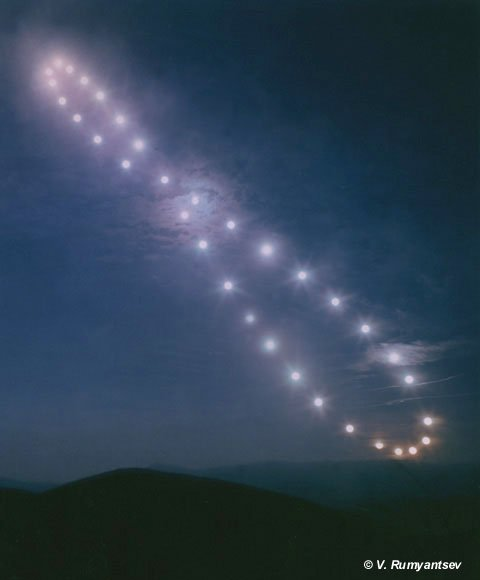Spanel Planetarium

# AnalemmaHave you ever noticed on some globes there is a strange figure 8, with tick marks and dates, usually printed somewhere in the middle of one of the oceans? It's called the analemma figure and here is what it is all about. The analemma shows the latitude on Earth where the Sun's rays are vertical on a given day of the year, and the "equation of time" which tells whether local clocks are ahead or behind solar time.

Equation of Time

The solar day is the length of time between one local noon, when the Sun crosses the meridian, to the next. But the length of the solar day is not always 24 hrs - its average is 24. The average is called Mean Time.

The Earth's axis is tilted which works to move the Sun's relative position north and south, hence the seasons. But the orbit around the Sun is elliptical instead of a circle so our planet moves faster at perihelion than at aphelion, which works to move the position of the Sun slightly east and west of the average position.

The north-south motion is the height of the figure 8 and the east-west motion is the width of the figure 8. The effects combine to create an everchanging offset between Mean Time and the appearance of local noon by as much as +/- 16 minutes. This difference is called "the equation of time."Photo Credit & Copyright: Vasilij Rumyantsev

Because of the equation of time, if you...

• take lots of pictures of the Sun
• each from the same location on Earth
• always at the same time of day
• at intervals for a whole year
• all on the same piece of film/file
This is the sort of image you will get.